# How to calculate the average rate of change in Excel?

If you want to calculate the average speed and how much time you will spend going from one place and another by bicycle, then you need to know the average speed when you drive a bike.

For an instance, consider you have recorded the time and distance at the time of traveling, now with that data, you can calculate the average bicycle speed – the average rate of change in an Excel sheet.

Follow the steps given below to calculate the average rate of change in an Excel sheet.

## Step 1

Open a Microsoft excel sheet and enter the data of time and distance as shown in the below screenshot for your reference. You can also change the time and distance as per your wish.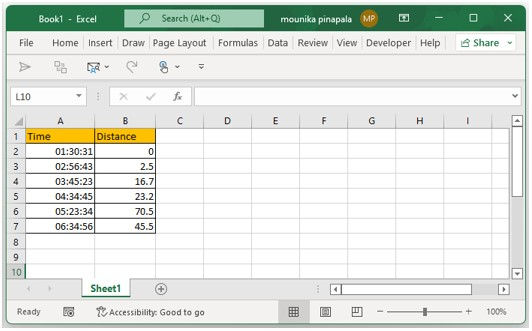## Step 2

Now you need to select a blank cell beside the cell with the last distance and enter the below given formula to calculate the average rate of change and press the enter key as shown in the below screenshot for your reference.

=(B7-B2)/((A7-A2)*24)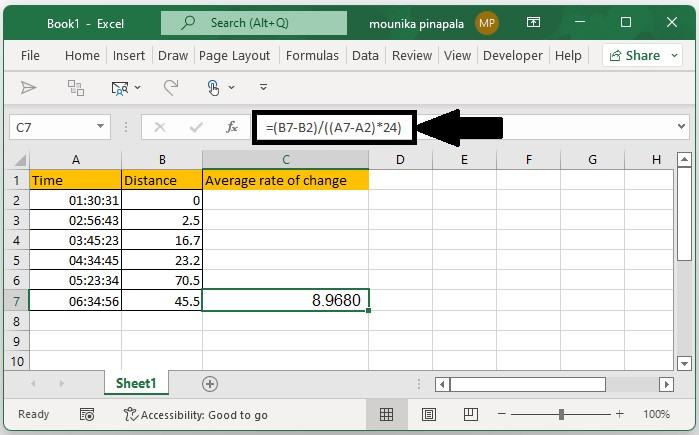## Step 3

Now you need to right click the enter formula cell and then select format cell from the list as shown in the below screenshot for your reference.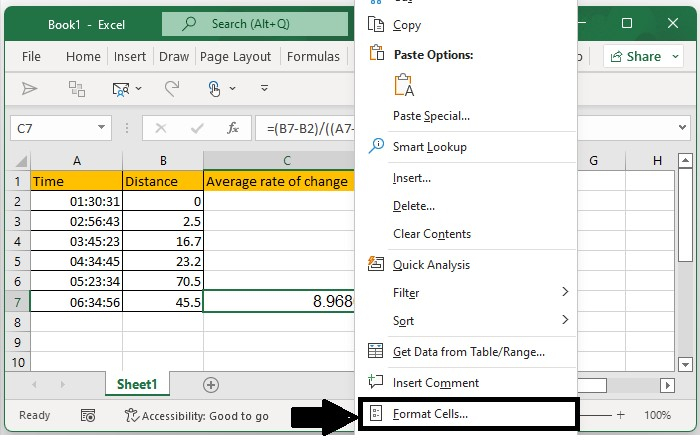## Step 4

Once you click on the format cell you will see a dialog box, in that select number from the category and then type 1 in decimal place, click on ok as shown in the below screenshot for your reference.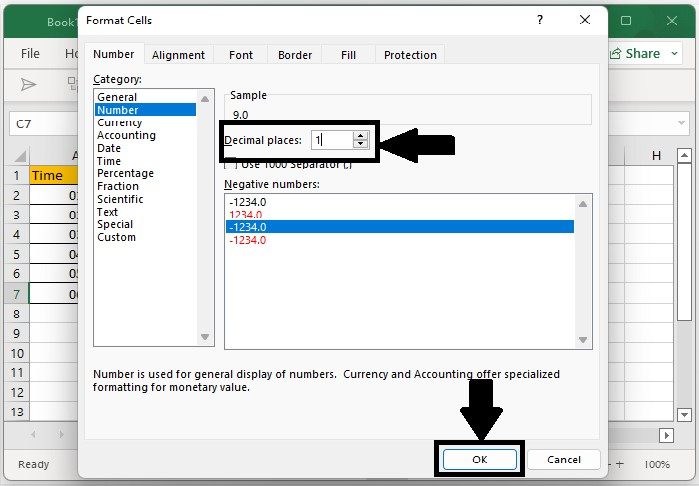Now you have calculated the average bicycle speed which is the average rate of change and format in excel. After formatting, you will see the result as 9.0 as shown in the below screenshot for your reference.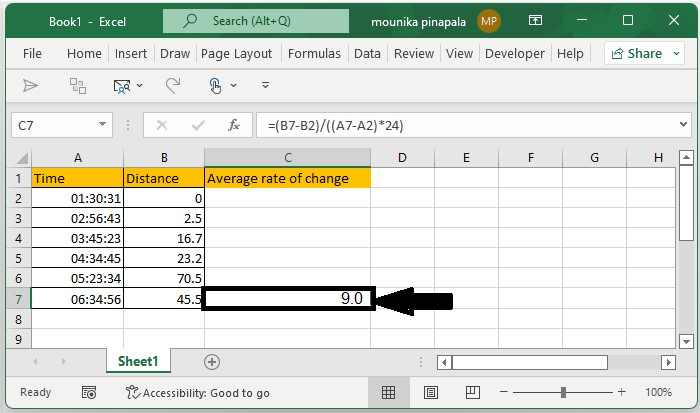Note − Not only format a cell using the format cell command by right-clicking the cell which as a formula, but you can also format the average bicycle speed by selecting the increase or decrease button on the home page. Kindly find the below screenshot for your reference.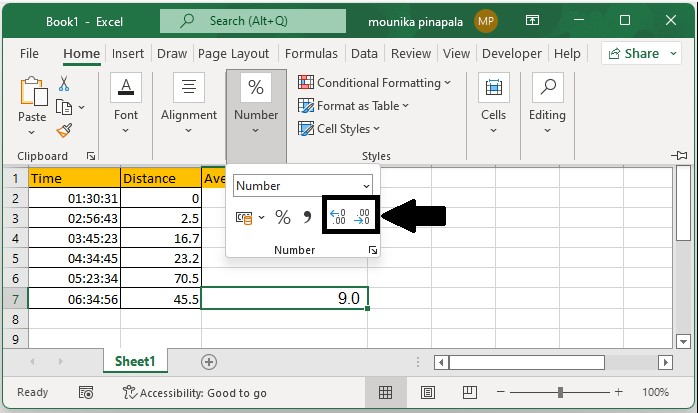## Conclusion

In this tutorial, we used a simple example to demonstrate how you can calculate the average rate of change in Excel.